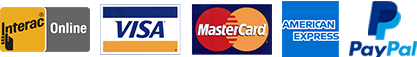# 360 Degree PWM Rotary Encoder Breakout

## Firgelli Automations

\$7.81
###### Payment Methods:###### Share:

360 Degree PWM Rotary Encoder Breakout

Description:

This type of switch is an incremental rotary encoder.  As a matter of fact, it is the most widely used of all rotary encoders due to its low cost and ability to provide signals that can be easily interpreted to provide motion related information such as velocity.

By rotating, the rotary encoder can count the output pulse frequency in positive and reverse direction during rotation, unlike a rotary potentiometer counter without counting limitation. With the button on the rotary encoder, it can be reset to its initial state starting from zero.

Testing code:

int redPin = 2;
int yellowPin = 3;
int greenPin = 4;
int aPin = 6;
int bPin = 7;
int buttonPin = 5;
int state = 0;
int longPeriod = 5000; // Time at green or red
int shortPeriod = 700; // Time period when changing
int targetCount = shortPeriod;
int count = 0;
void setup()
{
pinMode(aPin, INPUT);
pinMode(bPin, INPUT);
pinMode(buttonPin, INPUT);
pinMode(redPin, OUTPUT);
pinMode(yellowPin, OUTPUT);
pinMode(greenPin, OUTPUT);
}
void loop()
{
count++;
{
setLights(HIGH, HIGH, HIGH);
}
else
{
int change = getEncoderTurn();
int newPeriod = longPeriod + (change * 1000);
if (newPeriod >= 1000 && newPeriod <= 10000)
{
longPeriod = newPeriod;
}
if (count > targetCount)
{
setState();
count = 0;
}
}
delay(1);
}
int getEncoderTurn()
{

// return -1, 0, or +1
static int oldA = LOW;
static int oldB = LOW;
int result = 0;
int newA = digitalRead(aPin);
int newB = digitalRead(bPin);
if (newA != oldA || newB != oldB)
{
// something has changed
if (oldA == LOW && newA == HIGH)
{
result = -(oldB * 2 - 1);
}
}
oldA = newA;
oldB = newB;
return result;
}
int setState()
{
if (state == 0)
{
setLights(HIGH, LOW, LOW);
targetCount = longPeriod;
state = 1;
}
else if (state == 1)
{
setLights(HIGH, HIGH, LOW);
targetCount = shortPeriod;
state = 2;
}
else if (state == 2)
{
setLights(LOW, LOW, HIGH);
targetCount = longPeriod;
state = 3;
}
else if (state == 3)
{
setLights(LOW, HIGH, LOW);
targetCount = shortPeriod;
state = 0;
}
}
void setLights(int red, int yellow, int green)
{
digitalWrite(redPin, red);
digitalWrite(yellowPin, yellow);
digitalWrite(greenPin, green);